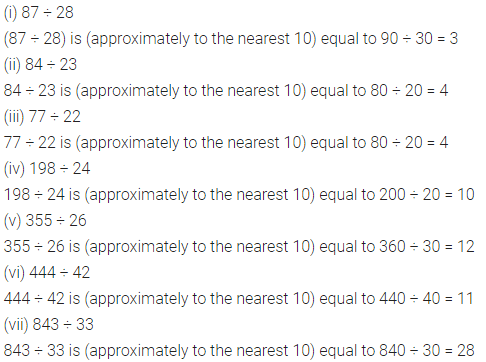# Selina Concise Mathematics Class 6 ICSE Solutions Chapter 2 Estimation

## Selina Concise Mathematics Class 6 ICSE Solutions Chapter 2 Estimation

Selina Publishers Concise Mathematics Class 6 ICSE Solutions Chapter 2 Estimation

### Estimation Exercise 2A – Selina Concise Mathematics Class 6 ICSE Solutions

Question 1.
Round off each of the following to the nearest ten :
(i) 62
(ii) 265
(iii) 543
(iv) 8261
(v) 6294
(vi) 3008
(vii) 72326
Solution:Question 2.
Round off each of the following to the nearest hundred :
(i) 748
(ii) 784
(iii) 2667
(iv) 5432
(v) 6388
(vi) 59237
Solution: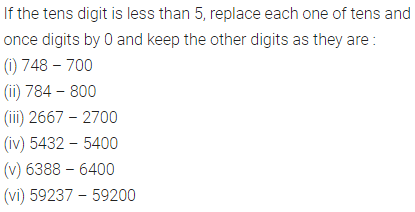Question 3.
Round off each of the following to the nearest thousand :
(i) 6475
(ii) 6732
(iii) 5352
(iv) 32568
(v) 9248
(vi) 83294
Solution: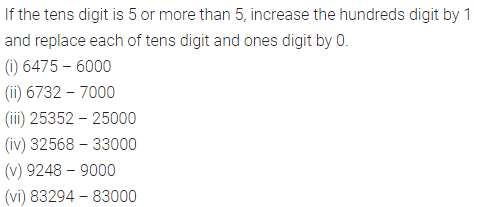Question 4.
Round off
(i) 578 to the nearest ten.
(ii) 578 to the nearest hundred.
(iii) 4327 to the nearest thousand.
(iv) 32974 to the nearest ten-thousand.
(v) 27487 to the nearest ten-thousand.
Solution:Question 5.
Round off each of the following to the nearest tens, hundreds and thousands.
(i) 864
(ii) 1249
(iii) 54, 547
(iv) 68, 076
(v) 56, 293
(vi) 7, 293
(vii) 89, 24, 379
Solution: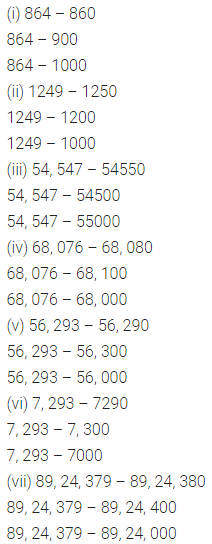Question 6.
Round off the following to the nearest tens ;
(i) ₹ 562
(ii) 837 m
(iii) 545 cm
(iv) ₹ 27
Solution:Question 7.
List all the numbers which can be round off to 30.
Solution: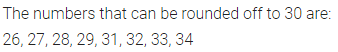Question 8.
List all the numbers which can be rounded off to 50.
Solution:Question 9.
Write the smallest and the largest numbers which are rounded off to 80.
Solution:Question 10.
Write the smallest and the largest numbers which are rounded off to 130.
Solution:### Estimation Exercise 2B – Selina Concise Mathematics Class 8 ICSE Solutions

Question 1.
Estimate the sum of each pair of numbers to the nearest ten :
(i) 67 and 44
(ii) 34 and 87
(iii) 23 and 66
(iv) 78 and 18
(v) 96 and 55
(vi) 76 and 62
(vii) 457 and 175
(viii) 474 and 173
(ix) 527 and 267
Solution:Question 2.
Estimate the sum of each pair of numbers to the nearest hundred :
(i) 336 and 782
(ii) 546 and 342
(iii) 270 and 495
(iv) 4280 and 5295
(v) 4230 and 2410
(vi) 30047 and 39287
Solution: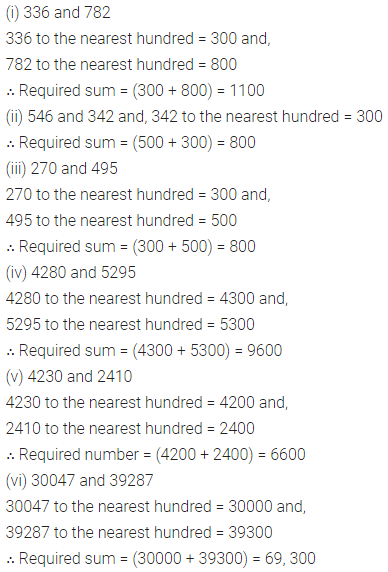Question 3.
Estimate the sum of the following pair of numbers to the nearest thousand:
(i) 53826 and 36455
(ii) 56802 and 22475
Solution:Question 4.
Estimate the following differences correct to nearest ten :
(i) 82 – 27
(ii) 96 – 36
(iii) 508 – 248
Solution: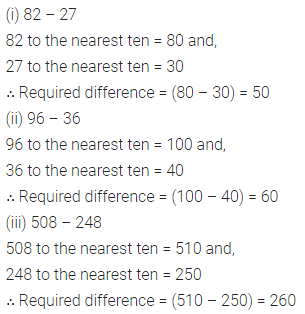Question 5.
Estimate each difference to the nearest hundred :
(i) 769 – 314
(ii) 856 – 687
(iii) 6352 – 2086
Solution: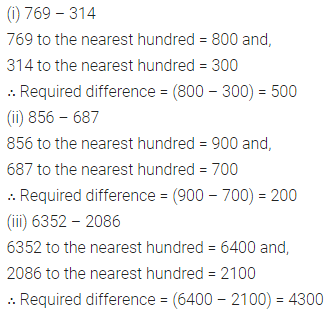Question 6.
Estimate each difference to the nearest thousand :
(i) 45974 – 38766
(ii) 76003 – 48399
Solution: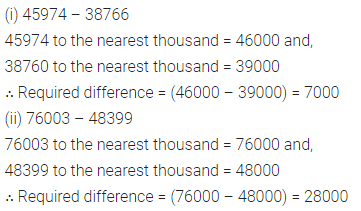Question 7.
Estimate each of the following products by rounding of each number to the nearest ten :
(i) 49 x 52
(ii) 63 x 38
(iii) 27 x 54
(iv) 53 x 85
(v) 74 x 67
(vi) 25 x 33
Solution:Question 8.
Estimate each of the following products by rounding off each number to the nearest hundred :
(i) 477 x 213
(ii) 624 x 236
(iii) 333 x 247
(iv) 537 x 283
(v) 382 x 127
(vi) 427 x 328
Solution: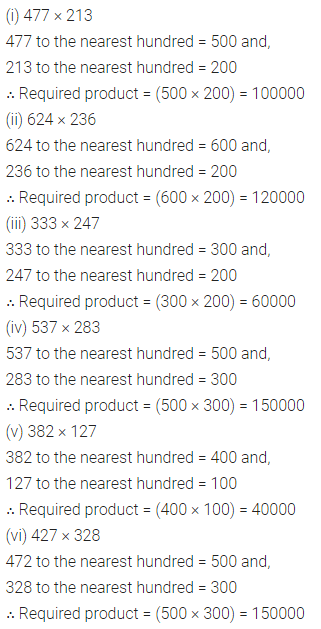Question 9.
Estimate each of the following products by rounding off the first number correct to nearest ten and the other number correct to nearest hundred :
(i) 28 x 287
(ii) 432 x 128
(iii) 48 x 165
(iv) 72 x 258
(v) 83 x 664
(vi) 44 x 250
Solution: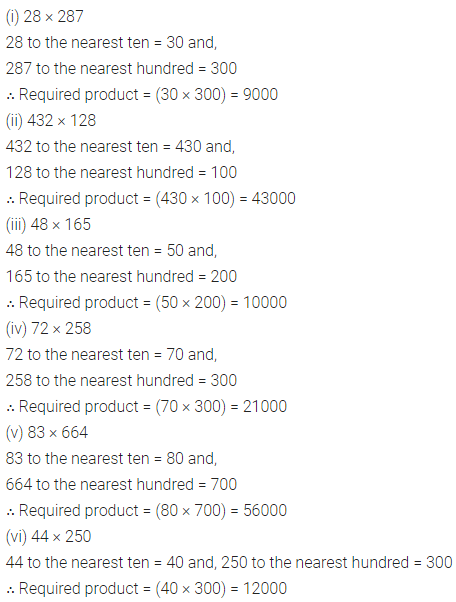Question 10.
Estimate each of the following quotients by converting each number to the nearest ten :
(i) 87 ÷ 28
(ii) 84 ÷ 23
(iii) 77 ÷ 22
(iv) 198 ÷ 24
(v) 355 ÷ 26
(vi) 444 ÷ 42
(vii) 843 ÷ 33
Solution: